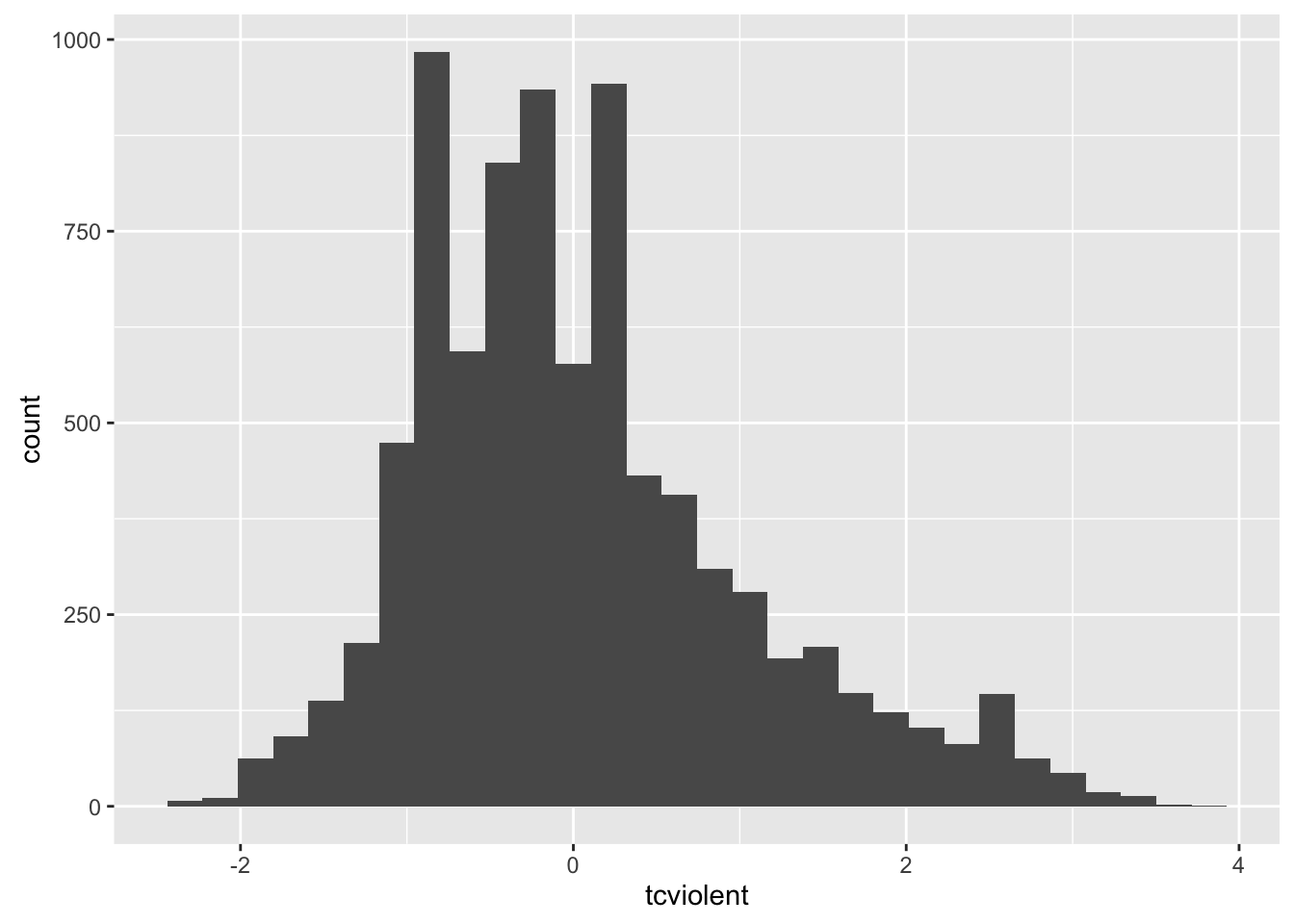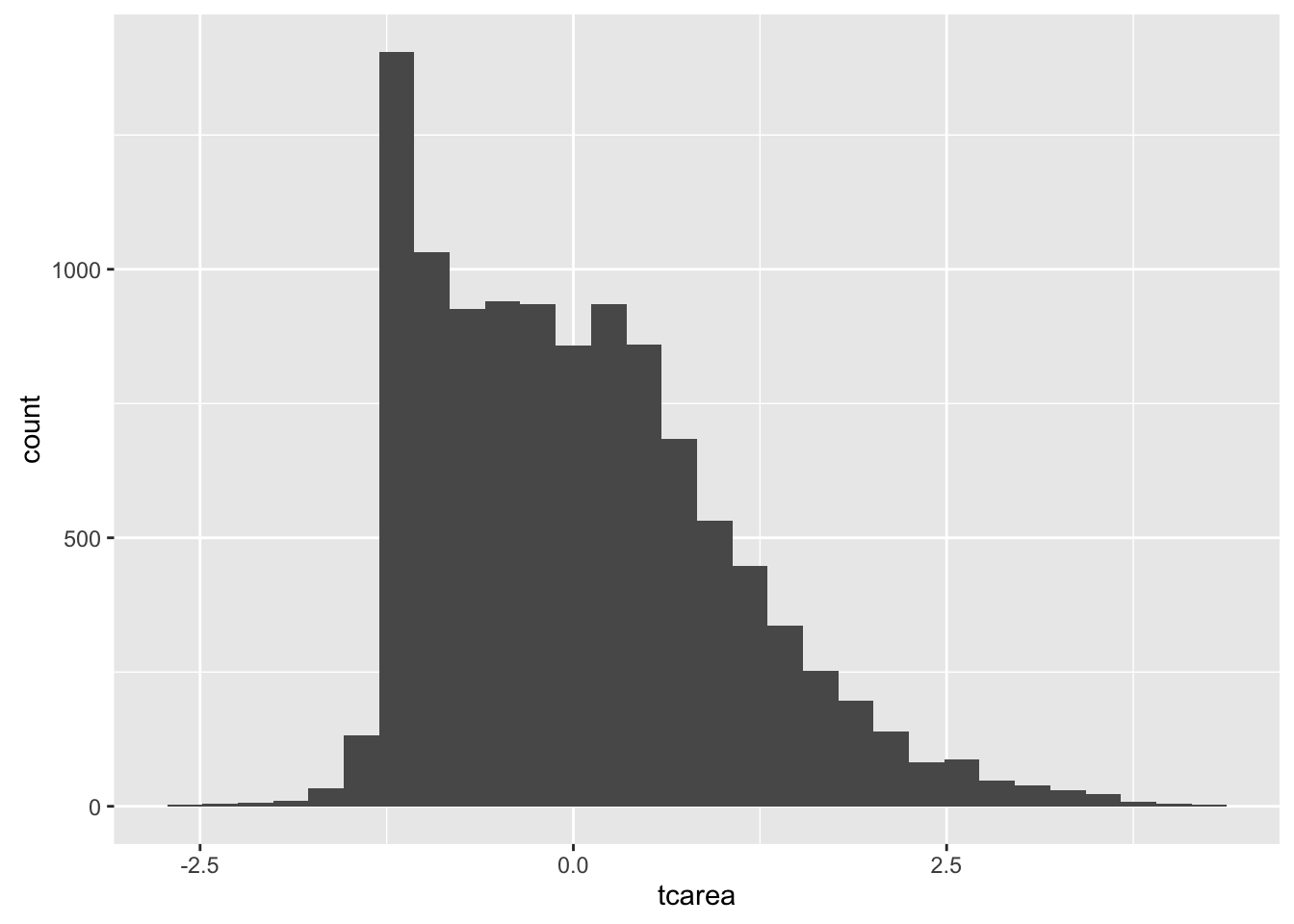## 6.1 Introduction

In this session we are going to cover regression analysis or, rather, we are beginning to talk about regression modelling. This form of analysis has been one the main technique of data analysis in the social sciences for many years and it belongs to a family of techniques called generalised linear models. Regression is a flexible model that allows you to “explain” or “predict” a given outcome (Y), variously called your outcome, response or dependent variable, as a function of a number of what is variously called inputs, features or independent, explanatory, or predictive variables (X1, X2, X3, etc.). Following Gelman and Hill (2007), I will try to stick for the most part to the terms outputs and inputs.

Today we will cover something that is called linear regression or ordinary least squares regression (OLS), which is a technique that you use when you are interested in explaining variation in an interval level variable. First we will see how you can use regression analysis when you only have one input and then we will move to situations when we have several explanatory variables or inputs.

We will return to the `BCS0708` data we used in previous sessions.

``````##R in Windows have some problems with https addresses, that's why we need to do this first:
urlfile<-'https://raw.githubusercontent.com/jjmedinaariza/LAWS70821/master/BCS0708.csv'
#We create a data frame object reading the data from the remote .csv file

We are going to look at the relationship between fear of violent crime (`tcviolent`), high scores represent high fear, with a variable measuring perceptions on antisocial behaviour in the local area (`tcarea`), high scores represent the respondents perceive a good deal of antisocial behaviour1:

``````library(ggplot2)
qplot(x = tcviolent, data = BCS0708)``````
``## `stat_bin()` using `bins = 30`. Pick better value with `binwidth`.``
``## Warning: Removed 3242 rows containing non-finite values (stat_bin).````qplot(x = tcarea, data = BCS0708)``
``## `stat_bin()` using `bins = 30`. Pick better value with `binwidth`.``
``## Warning: Removed 677 rows containing non-finite values (stat_bin).``We can see both are skewed. Let’s look at the scatterplot:

``````ggplot(BCS0708, aes(x = tcarea, y = tcviolent)) +
geom_point(alpha=.2, position="jitter") ``````
``## Warning: Removed 3664 rows containing missing values (geom_point).``